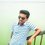# Prove this derivative of the Pythagorean Theorem using calculus, trigonometry, geometry, or plain old algebra, which yields the shortest, simplest proofs!

Prove that for a, b, c > 0, if a² + b² = c², then a + b <= c sqrt(2) ANSWER: BY

Calculus: The heavy hand of calculus does not provide a short or elegant proof, but I always like seeing more than one approach.

a² + b² = c² a² = c² - b² a = sqrt(c² - b²) a + b = sqrt(c² - b²) + b

To maximize a + b, we take its derivative and set it to 0. Derivative is (sqrt(c² - b²) - b) / sqrt(c² - b²) Setting that to 0, we have sqrt(c²-b²) = b c² - b² = b² c² = 2b² We can also reach this point by seeing that the derivative is also equal to (a -b) / a Setting a - b = 0, we have a = b, b = a = c/sqrt(2) = sqrt(2) c/2 At the maximum, a + b = sqrt(2) c, therefore a + b <= sqrt(2) c.

Trigonometry: a= c cos t and b = c sin t so a + b = c ( cos t + sin t) As c is constant, we need to maximise cos t + sin t This is of the form A cos t + B sin t A = 1 and B =1 let A = 1 =r cos a B = 1 = r sin a square and add: r = sqrt(2) and tan a = 1 so 1 cos t + 1 sin t = r cos a cos t + r sin a sin t = r cos(t-a) = sqrt(2) cos(t-pi/4) a+b = sqrt(2) cos(t-pi/4) c so <= sqrt(2) C as cos(t-pi/4) <= 1 (I'm not sure I followed all that though.) And the simplest proofs follow.

Geometry: a,b,c are the sides of a right triangle. Since a, b, and c are positive real numbers: a+b > sqrt(2) c => (a+b)² > 2c² => 2ab > c². (since a² + b² = c²)

The triangle ABC is a right triangle, so it can be inscribed in a circle with diameter c.

The height of this triangle, h, <= c/2 (radius of circle).

The area of triangle = ch/2 = ab/2.

h <= c/2 (radius of circle). h² = ch/2 <= (c/2)² = c²/4 So ab/2 <= c²/4 and 2ab <= c²

Algebra We obviously have that: 0 <= (a - b)² 0 <= a² - 2ab + b² a² + 2ab + b² <= 2a² + 2b² = 2c² (a + b)² <= 2c²

EDIT: I should have just written: (a + b)² <= (a - b)² + (a + b)² = 2c² It would have been a nice one liner lol

(a-b)² >= 0 since a square is always >= 0 a² - 2ab + b² >= 0 a² + b² >= 2ab 2a² + 2b² >= a² + 2ab + b² (add a² + b² to both sides) 2c² >= (a+b)² (using a² + b² = c²) sqrt(2) c >= a+b since a,b,c>06 years, 7 months ago

This discussion board is a place to discuss our Daily Challenges and the math and science related to those challenges. Explanations are more than just a solution — they should explain the steps and thinking strategies that you used to obtain the solution. Comments should further the discussion of math and science.

When posting on Brilliant:

• Use the emojis to react to an explanation, whether you're congratulating a job well done , or just really confused .
• Ask specific questions about the challenge or the steps in somebody's explanation. Well-posed questions can add a lot to the discussion, but posting "I don't understand!" doesn't help anyone.
• Try to contribute something new to the discussion, whether it is an extension, generalization or other idea related to the challenge.

MarkdownAppears as
*italics* or _italics_ italics
**bold** or __bold__ bold
- bulleted- list
• bulleted
• list
1. numbered2. list
1. numbered
2. list
Note: you must add a full line of space before and after lists for them to show up correctly
paragraph 1paragraph 2

paragraph 1

paragraph 2

[example link](https://brilliant.org)example link
> This is a quote
This is a quote
    # I indented these lines
# 4 spaces, and now they show
# up as a code block.

print "hello world"
# I indented these lines
# 4 spaces, and now they show
# up as a code block.

print "hello world"
MathAppears as
Remember to wrap math in $$ ... $$ or $ ... $ to ensure proper formatting.
2 \times 3 $2 \times 3$
2^{34} $2^{34}$
a_{i-1} $a_{i-1}$
\frac{2}{3} $\frac{2}{3}$
\sqrt{2} $\sqrt{2}$
\sum_{i=1}^3 $\sum_{i=1}^3$
\sin \theta $\sin \theta$
\boxed{123} $\boxed{123}$

Sort by:

This is a simple problem of finding the maximum value of $f(a,b)=a+b$ subject to the constraint $a^{2}+b^{2}=c^{2}$ . The method of Lagranges multipliers gives the answer $a+b \leq c \sqrt{2}$

- 6 years, 7 months ago